# 1.2. Data models

A data model is a model that describes in an abstract way how data are represented in a business organization, an information system or a database management system - Wikipedia
[Section notes PDF 310Kb].

# 1.2.01. Introduction

• Relational model
• Abstract operations on relations
• Set theoretic operations
• Relational-specific operations
• Basic algebra operations
• Union, Intersection, Difference
• Cross product

# 1.2.02. Relational model

• Table as relation
• Row as tuple
• real world entity or relationship
• fact
• Column as attribute
• Domain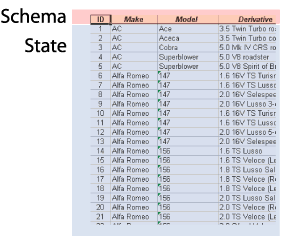The concept of a relation is abstract, therefore we have a number of different ways of visualising it.

# 1.2.03. Relation

• Relation schema R(A1, A2, A3.. An)
• Design side
• Assertion/declaration
• Relation state
• Data side
• set of n-tuples
• each one an ordered list of values
• 1NF: each value is atomic, no composite/multivalue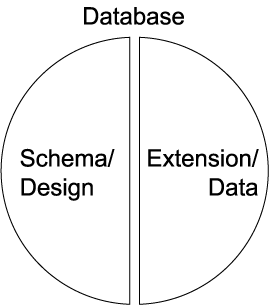# 1.2.04. Abstract operations

• Database lifecycle
• design, populate, evolve
• Insert
• tuple (a1,a2,a3…an)
• Delete
• tuple (a1,a2,a3…an)
• Update (or modify)
• tuple (a1,a2,a3…an)
• attribute to change, new value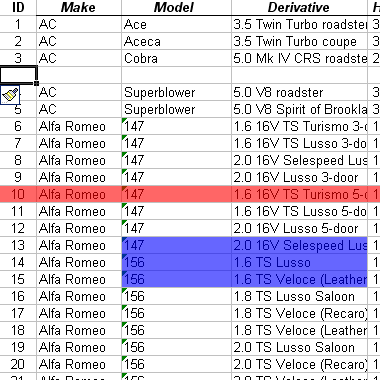All the operations described in the next few sections are abstract. We're going to see how valuable they can be in processing real world data later.

# 1.2.05. Basic algebra

• Two categories
• Set theoretic operations
• Union, Intersection etc.
• Relational specific
• Select, project and join

At this stage we're talking about set theoretical operators on the Relational model, not SQL instructions which confusingly have identical names and only similar behaviour.

# 1.2.06. Select operation

• SELECT a subset of tuples from a relation
• Uses selection condition
• Evaluate each tuple to true of false
• Sigma (s)
• output = s(cond)(input_relation)
• Relation schema: R(output) = R(input_relation)
• Commutative

# 1.2.07. Project operation

• PROJECT a subset of attributes for all tuples from a relation
• Pi (p)
• p<attribute list>(R)
• If sublist is only non-key attributes
• might get duplicates
• Removes duplicates
• Attribute list:sublist example

The result set of the operation is itself a relational. That output relation will contain the same number of rows as the input, however it may contain a different number of columns; fewer if a subset of attributes is projected; more if derived or aggregated attributes are included.

# 1.2.07. Sequences of operations

• Select followed by projection
• Area clipping: rows then columns
• p<attr list>
(
s(select_cond)(R))
• Rename operation (r)
• Renames attributes list2 from list1
• r(new_attr_names)(R)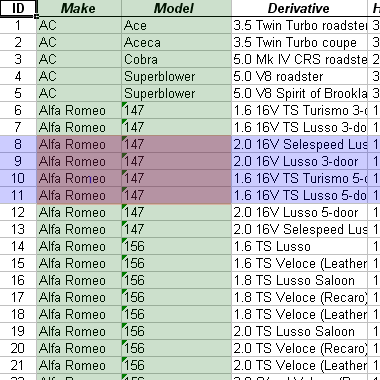# 1.2.08. Rename operation

• Attribute renaming only
• Cannot alter domain, or add/remove attr
• Rename operation (r)
• Renames attributes list2 from list1
• r(new_attr_names)(R)
• Implicit renaming
• Order dictated by relational schema

# 1.2.08. Set Theoretic

• Binary operation: two relations
• Sets of tuples
• Union compatibility (same attributes)
• Union (R u S)
• Intersection (R n S)
• Commutative (R u (S u T) = (R u S) u T)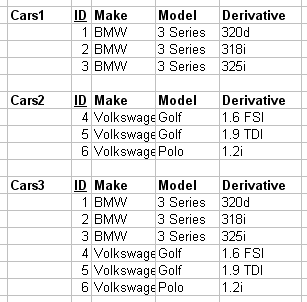# 1.2.08b. Set difference

• Set difference
• Non-commutative (R-S != S-R)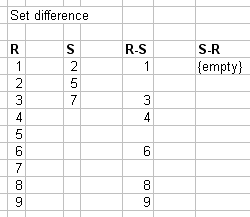# 1.2.09. Cross product

• Cartesian product of two relations
• R x S
• Also known as
• Cross product
• Cross join
• Cross product diagram
• Introduction to complexity
• Computationally explosive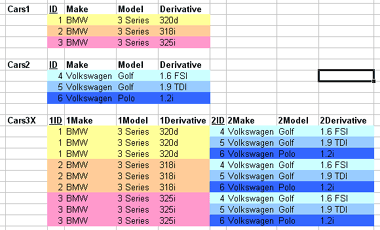# 1.2.10. Relational algebra/model notation

• Relational schema R(A1, A2,…,An)
• Relation state r or r(R)
• Set of unordered tuples
• r = {t1, t2,…,tn}
• Each n-tuple is an ordered list of values
• t = <v1, v2,…,vn>
• ith value in t = vi called t[Ai]
• r(R) subset of (dom(A1) x dom(A2)... x dom(An))

# 1.2.11. Constraints

• Domain constraint
• For all v in t of r(R)
vi is an element of dom(Ai)
• Entity constraint
• K = SKmin
• t[K] != null
• Key constraint
• Superkey SK as identifying subset of attributes
• t1[SK] != t2[SK]

# 1.2.12. Referential integrity

• Given two relations R1 and R2
• R1 contains a foreign key (FK) that references
• A primary key (PK) in R2
• R1 referencing relation, R2 referenced relation
• Shared domains: dom(FK) = dom(PK)
• Foreign exists: t1 in r(R1), t2 in r(R2)
• t1[FK] = t2[PK] || NULL